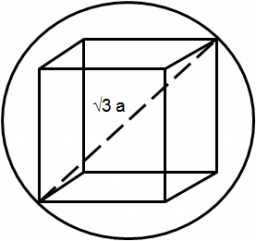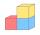# Cube in a sphere

The cube is inscribed in a sphere with a volume 7253 cm3. Determine the length of the edges of a cube.

a =  13.9 cm

### Step-by-step explanation:Did you find an error or inaccuracy? Feel free to write us. Thank you!Math student
i am good at this

3 years ago  2 LikesCrazy Butterfly
This is easy.Wiggly
Maths is good.Tips to related online calculators
Need help to calculate sum, simplify or multiply fractions? Try our fraction calculator.
Do you want to convert length units?
Tip: Our volume units converter will help you with the conversion of volume units.
Pythagorean theorem is the base for the right triangle calculator.

#### You need to know the following knowledge to solve this word math problem:

We encourage you to watch this tutorial video on this math problem:

## Related math problems and questions:

• Cube in sphereThe cube is inscribed in a sphere with a radius r = 6 cm. What percentage is the volume of the cube from the volume of the ball?
• Cube in sphereThe sphere is an inscribed cube with an edge of 8 cm. Find the sphere's radius.
• Cube diagonalsDetermine the volume and surface area of the cube if you know the length of the body diagonal u = 216 cm.
• Body diagonal - cubeCalculate the surface and cube volume with body diagonal 15 cm long.
• CalculateCalculate the length of the wall diagonal of the cube with an edge 5 cm long.
• Cube diagonalsCalculate the length of the side and the diagonals of the cube with a volume of 27 cm3.
• CuboidCuboid with edge a=6 cm and space diagonal u=31 cm has volume V=900 cm3. Calculate the length of the other edges.
• Cube diagonalsThe cube has a wall area of 81 cm square. Calculate the length of its edge, wall, and body diagonal.
• Cube and sphereCube with the surface area 150 cm2 is described sphere. What is sphere surface?
• Sphere vs cubeHow many % of the surface of a sphere of radius 12 cm is the surface of a cube inscribed in this sphere?
• Cuboid easyThe cuboid has the dimensions a = 12 cm, b = 9 cm, c = 36 cm. Calculate the length of the body diagonal of the cuboid.
• Body diagonalCuboid with base 7cm x 3,9cm and body diagonal 9cm long. Find the height of the cuboid and the length of the diagonal of the base,
• Cube diagonalDetermine the length of the cube diagonal with edge 37 mm.The height of a regular quadrilateral prism is v = 10 cm, the deviation of the body diagonal from the base is 60°. Determine the length of the base edges, the surface, and the volume of the prism.Calculate the length of wall diagonal of the cube whose surface is 384 cm square.Calculate the cube volume, whose body diagonal size is 75 dm. Draw a picture and highlight the body diagonal.The prism with the edges of the lengths x cm, 2x cm, and 3x cm has volume 20250 cm3. What is the area of the surface of the prism?